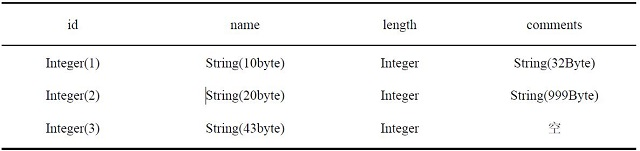ET大脑

# 表格存储的数据存储• 对于 id=1 的行，其数据大小为：len (‘id’) + len (‘name’) + len (‘length’) + len (‘comments’) = 8 Byte + 10 Byte + 8 Byte + 32 Byte = 78 Byte。

• 对于 id=2 的行，其数据大小为：len (‘id’) + len (‘name’) + len (‘length’) + len (‘comments’) = 8 Byte + 20 Byte + 8 Byte+ 999 Byte = 1055 Byte。

• 对于 id=3 的行，其数据大小为：len (‘id’) + len (‘name’) + len (‘length’) = 8 Byte + 43 Byte + 8 Byte = 71 Byte。

• 因此，表的数据大小之和为： 78 + 1055 + 71 =1204 Byte。